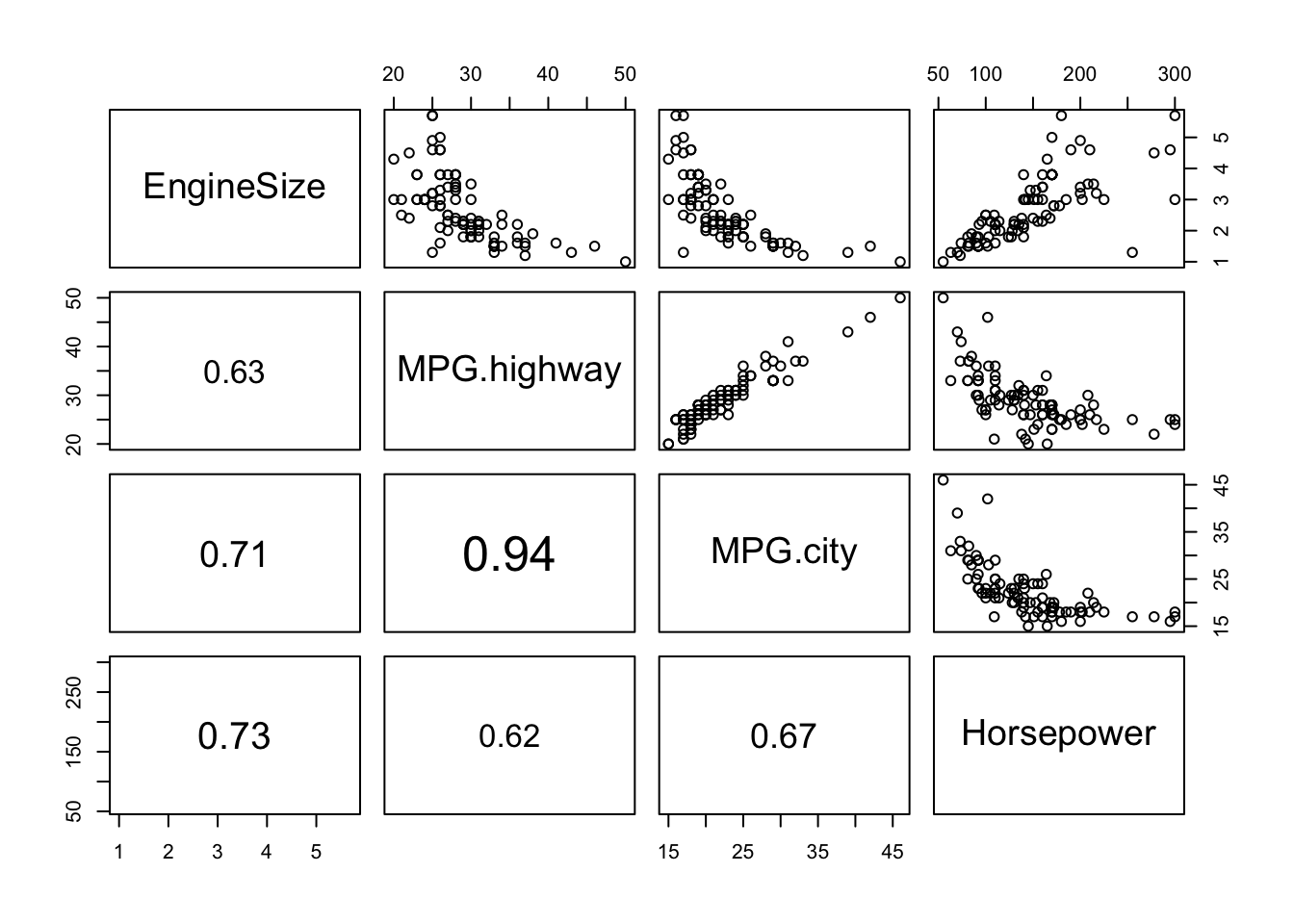### Learning objectives

In today’s Lab you will gain practice with the following concepts from today’s class:

• Interpreting linear regression coefficients of numeric covariates
• Interpreting linear regression coefficients of categorical variables
• Applying the “2 standard error rule” to construct approximate 95% confidence intervals for regression coefficients
• Using the `confint` command to construct confidence intervals for regression coefficients
• Using `pairs` plots to diagnose collinearity
• Using the `update` command to update a linear regression model object
• Diagnosing violations of linear model assumptions using `plot`

``library(tidyverse)``
``## ── Attaching packages ──────────────────────────────────────────────────────── tidyverse 1.2.1 ──``
``````## ✔ ggplot2 3.3.2     ✔ purrr   0.3.3
## ✔ tibble  2.1.3     ✔ dplyr   0.8.3
## ✔ tidyr   1.0.0     ✔ stringr 1.4.0
## ✔ readr   1.3.1     ✔ forcats 0.4.0``````
``## Warning: package 'ggplot2' was built under R version 3.6.2``
``````## ── Conflicts ─────────────────────────────────────────────────────────── tidyverse_conflicts() ──
``````library(knitr)

Cars93 <- as_tibble(MASS::Cars93)
# If you want to experiment with the ggpairs command,
# you'll want to run the following code:
# install.packages("GGally")
# library(GGally)``````

### Linear regression with Cars93 data

(a) Use the `lm()` function to regress Price on: EngineSize, Origin, MPG.highway, MPG.city and Horsepower.

``````cars.lm <- lm(Price ~ EngineSize + Origin + MPG.highway + MPG.city + Horsepower,
data = Cars93)``````

(b) Use the `kable()` command to produce a nicely formatted coefficients table. Ensure that values are rounded to an appropriate number of decimal places.

``kable(summary(cars.lm)\$coef, digits = c(3, 3, 3, 4), format = "markdown")``
Estimate Std. Error t value Pr(>|t|)
(Intercept) 3.470 6.224 0.558 0.5786
EngineSize 1.458 1.043 1.398 0.1656
Originnon-USA 4.714 1.393 3.385 0.0011
MPG.highway -0.006 0.346 -0.017 0.9868
MPG.city -0.252 0.367 -0.687 0.4939
Horsepower 0.109 0.019 5.808 0.0000

(c) Interpret the coefficient of `Originnon-USA`. Is it statistically significant?

The coefficient of `Originnon-USA` is 4.71. This indicates that, all else in the model held constant, vehicles manufactured outside of the USA carry a price tag that is on average \$4.71 thousand dollars higher than vehicles manufactered in the US. The coefficient is statistically significant at the 0.05 level.

(d) Interpret the coefficient of `MPG.highway`. Is it statistically significant?

The coefficient of `MPG.highway` is -0.006, which is close to 0 numerically and is not statistically significant. Holding all else in the model constant, MPG.highway does not appear to have much association with Price.

(d) Use the “2 standard error rule” to construct an approximate 95% confidence interval for the coefficient of `MPG.highway`. Compare this to the 95% CI obtained by using the `confint` command.

``````est <- coef(cars.lm)["MPG.highway"]
se <- summary(cars.lm)\$coef["MPG.highway", "Std. Error"]
# 2se rule confidence interval:
c(est - 2 *se, est + 2 * se)``````
``````## MPG.highway MPG.highway
##  -0.6983730   0.6868413``````
``````# confint approach
confint(cars.lm, parm = "MPG.highway")``````
``````##                  2.5 %  97.5 %
## MPG.highway -0.6940817 0.68255``````

We see that the confidence intervals obtained via the two different approaches are essentially identical.

(e) Run the `pairs` command on the following set of variables: EngineSize, MPG.highway, MPG.city and Horsepower. Display correlations in the Do you observe any collinearities?

``````panel.cor <- function(x, y, digits = 2, prefix = "", cex.cor, ...)
{
usr <- par("usr"); on.exit(par(usr))
par(usr = c(0, 1, 0, 1))
r <- abs(cor(x, y))
txt <- format(c(r, 0.123456789), digits = digits)
txt <- paste0(prefix, txt)
if(missing(cex.cor)) cex.cor <- 0.4/strwidth(txt)
text(0.5, 0.5, txt, cex = pmax(1, cex.cor * r))
}

pairs(Cars93[,c("EngineSize", "MPG.highway", "MPG.city", "Horsepower")],
lower.panel = panel.cor)``````The MPG.highway and MPG.city variables are very highly correlated.

(f) Use the `update` command to update your regression model to exclude `EngineSize` and `MPG.city`. Display the resulting coefficients table nicely using the `kable()` command.

``cars.lm2 <- update(cars.lm, . ~ . - EngineSize - MPG.city)``
``kable(summary(cars.lm2)\$coef, digits = c(3, 3, 3, 4), format = "markdown")``
Estimate Std. Error t value Pr(>|t|)
(Intercept) 7.513 5.705 1.317 0.1913
Originnon-USA 3.484 1.208 2.885 0.0049
MPG.highway -0.287 0.145 -1.986 0.0501
Horsepower 0.130 0.015 8.946 0.0000

(g) Does the coefficient of `MPG.highway` change much from the original model? Calculate a 95% confidence interval and compare your answer to part (d). Does the CI change much from before? Explain.

``````# old
confint(cars.lm, parm = "MPG.highway")``````
``````##                  2.5 %  97.5 %
## MPG.highway -0.6940817 0.68255``````
``````# new
confint(cars.lm2, parm = "MPG.highway")``````
``````##                  2.5 %       97.5 %
## MPG.highway -0.5741476 0.0001540153``````

Both the estimate and the confidence interval change greatly. When we remove the highly collinear MPG.city variable from the model, the coefficient of MPG.highway increases (in magnitude). We also get a much narrower confidence interval, indicating that we are able to more precisely estimate the coefficient of MPG.highway.

(h) Run the `plot` command on the linear model you constructed in part (f). Do you notice any issues?

``````par(mfrow = c(2, 2))
plot(cars.lm2)``````